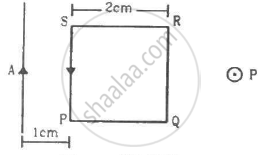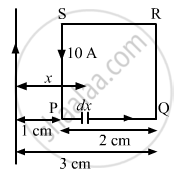Advertisement Remove all ads

# A Square Loop Pqrs Carrying a Current of 6.0 a is Placed Near a Long Wire Carrying 10 a as Shown in Figure. (A) Show that the Magnetic Force Acting on the Part Pq is Equal and Opposite to the Part Rs. - Physics

Short Note

A square loop PQRS carrying a current of 6.0 A is placed near a long wire carrying 10 A as shown in figure. (a) Show that the magnetic force acting on the part PQ is equal and opposite to the part RS. (b) Find the magnetic force on the square loop.Advertisement Remove all ads

#### Solution

Given:
Current in the loop, i1 = 6 A
Current in the wire, i2 = 10 A

Now, consider an element on PQ of width dx at a distance x from the wire.Force on the element is given by $dF = \frac{\mu_0 i_1 i_2}{2\pi x}dx$

Force acting on part PQ is given by

$F_{PQ} = \frac{\mu_0 i_1 i_2}{2\pi} \int\limits_1^3 \frac{dx}{x}$

$= 2 \times {10}^{- 7} \times 6 \times 10[\text{ ln } x {]_1}^3$

$= 120 \times {10}^{- 7} \text{ ln } \left( 3 \right) N$

$\text{ Similarly },$

$F_{RS} = \frac{\mu_0 i_1 i_2}{2\pi} \int\limits_3^1 \frac{dx}{x}$

$= 120 \times {10}^{- 7} \text{ ln } \left( \frac{1}{3} \right)$

$= - 120 \times {10}^{- 7} \text{ ln } \left( 3 \right) N$

Both forces are equal in magnitude, but they are opposite in direction.

(b) The magnetic field intensity due to wire on SP is given by

$B = \frac{\mu_0 i_2}{2\pi r}$
Force on part SP is given by

$F_{SP} = i_1 Bl$

$= \frac{\mu_0 i_1 i_2}{2\pi}\left( \frac{l}{r} \right)$

$= \frac{\mu_0 i_1 i_2}{2\pi}\left( \frac{2}{1} \right)$

(Towards right)

Force on part RQ is given by

$F_{RQ} = i_1 Bl$
$= \frac{\mu_0 i_1 i_2}{2\pi}\left( \frac{l}{r} \right)$
$= \frac{\mu_0 i_1 i_2}{2\pi}\left( \frac{2}{3} \right)$

(Towards left)

Thus, the net force on the loop is given by

$F_{net} = F_{SP} - F_{RQ}$

$= \frac{\mu_0 i_1 i_2}{2\pi}\left( \frac{2}{1} - \frac{2}{3} \right)$

$= 2 \times {10}^{- 7} \times 6 \times 10 \times \frac{4}{3}$

$= 16 \times {10}^{- 6}$  N

(Towards right)

Concept: Torque on a Current Loop in Magnetic Field
Is there an error in this question or solution?
Advertisement Remove all ads

#### APPEARS IN

HC Verma Class 11, Class 12 Concepts of Physics Vol. 2
Chapter 13 Magnetic Field due to a Current
Q 31 | Page 251
Advertisement Remove all ads

#### Video TutorialsVIEW ALL 

Advertisement Remove all ads
Share
Notifications

View all notifications

Forgot password?